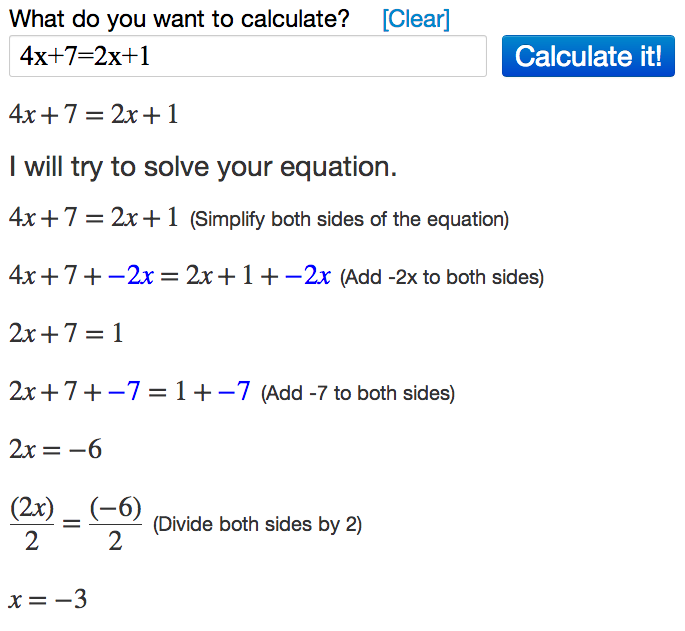# Solving Equations Using Algebra Calculator

Learn how to use the Algebra Calculator to solve equations.

### Example Problem

Solve the following equation for x:
4x+7=2x+1

### How to Solve the Equation in Algebra Calculator

First go to the Algebra Calculator main page.

Type the following:

1. 4x+7=2x+1
Try it now: 4x+7=2x+1

### Clickable Demo

Try entering 4x+7=2x+1 into the text box.After you enter the expression, Algebra Calculator will solve the equation 4x+7=2x+1 for x to get x=-3.### More Examples

Here are more examples of how to solve equations in Algebra Calculator. Feel free to try them now.